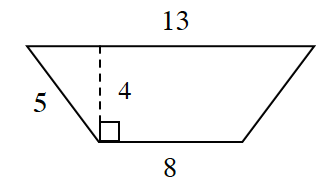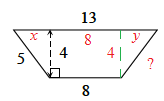### Home > INT2 > Chapter 9 > Lesson 9.1.4 > Problem9-47

9-47.Use what you know about similar figures to complete the following tasks.

1. What are the area and perimeter of the trapezoid at right?

Do not assume the trapezoid is isosceles even though it looks like it.
Start by solving for $x$ in the diagram, then solve for $y$, then calculate the length of the unknown side of the trapezoid.Area $= 42$ square units

2. What are the area and perimeter of a trapezoid that is similar to this one but reduced by a linear scale factor of $\frac { 1 } { 3 }$?